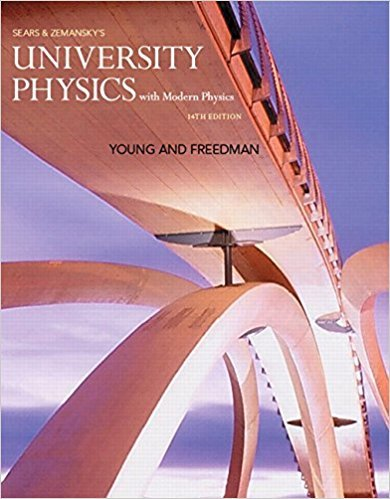×
×

# Answer: Under what conditions does the magnitude of theISBN: 9780321973610 228

## Solution for problem Q2.6 Chapter 2

University Physics with Modern Physics (1) | 14th Edition

• Textbook Solutions
• 2901 Step-by-step solutions solved by professors and subject experts
• Get 24/7 help from StudySoup virtual teaching assistantsUniversity Physics with Modern Physics (1) | 14th Edition

4 5 1 296 Reviews
25
4
Problem Q2.6

Under what conditions does the magnitude of the average velocity equal the average speed?

Step-by-Step Solution:
Step 1 of 3

Goals • To study electric charge and charge conservation • To see how objects become charged • To calculate the electric force between objects using Coulomb’s law • To learn the distinction between electric force and electric field • To calculate the electric field due to many charges • To visualize and interpret electric fields Introduction • Water makes life possible...

Step 2 of 3

Step 3 of 3

##### ISBN: 9780321973610

This full solution covers the following key subjects: . This expansive textbook survival guide covers 44 chapters, and 4574 solutions. Since the solution to Q2.6 from 2 chapter was answered, more than 252 students have viewed the full step-by-step answer. This textbook survival guide was created for the textbook: University Physics with Modern Physics (1), edition: 14. The answer to “Under what conditions does the magnitude of the average velocity equal the average speed?” is broken down into a number of easy to follow steps, and 14 words. The full step-by-step solution to problem: Q2.6 from chapter: 2 was answered by , our top Physics solution expert on 01/09/18, 07:46PM. University Physics with Modern Physics (1) was written by and is associated to the ISBN: 9780321973610.

Unlock Textbook Solution